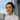# Bubble sort explained

December 16, 2017Photo by Sime Basioli on Unsplash

The goal of bubble sort is to sort an array in ascending or descending order according to a sortable property.

In our example below, we just use an array of integers to directly simulate the sortable property (a number), but in reality, you can imagine that that probably isn’t a frequent use case. What’s probably more common is sorting an array of objects by one of their properties, like IDs.

Before diving in, here’s a quick low down on the effectiveness of bubble sort:

• Quadratic time complexity, or `O(n^2)`, which means it can be very slow when working on a large input array
• Constant space complexity, or `O(1)`, which is a perfect score

Bubble sort certainly does not exude the kind of “ooh this is such a clever algorithm” aura that other sorting algorithms like merge sort might have, but it’s nonetheless an algorithm that works OK for small inputs.

We’ll expand on time and space complexity of the algorithm soon. But first, let’s look at how bubble sort is implemented.

## Bubble sort in JavaScript

Here’s my implementation of bubble sort in JavaScript. Full disclosure, I haven’t cross-checked my implementation with “model implementations” that are probably available on the internet. I understood conceptually how bubble sort was supposed to work, and implemented it in a way that made sense to me in JavaScript (and verified that it works as intended).

In plain English, here’s the sequence of operations:

1. Starting from the back of the array, point at the last element
2. Compare that element with the one to its left

• if current value is smaller than the one to its left, swap them (so the smaller value is now correctly in front of the larger value)
• if current value is larger than the one to its left, do nothing (they are already in their right places relative to each other)
3. Shift pointer one step left
4. Repeat steps 2 and 3 until we hit the first element in the array, which will not have an element to its left
5. That first element is now considered properly sorted, and can be ignored from now on
6. Reset the pointer to point at the last element in the array once again
7. Repeat steps 1-6 above until the only element left unsorted is the last element, at which point, that final element can implicitly be considered as sorted relative to the rest of the elements in the array
``````/**
* Sort an array in ascending order.
* Delete null values before output.
* @param {number[]} array
* @return {number[]}
*/
function bubbleSort (array) {
var count = 0
var length = array.length

// traverse the array (length of array) times
while (count !== length) {
for (var i = length; i > count; i--) {
var current = array[i]
var left = array[i - 1]

// delete element if value is null
if (current === null) {
array.splice(i, 1)
continue
}

// swap
if (left !== undefined && left > current) {
array[i - 1] = current
array[i] = left
}
}
count++
}
return array
}

// test with positive numbers only
console.log(bubbleSort([109, 90, 44, 39, 4, 24, 2, 0, 10]))
// => [ 0, 2, 4, 10, 24, 39, 44, 90, 109 ]

// test with negative numbers
console.log(bubbleSort([10, 9, 8, 7, 6, 4, 5, 3, 2, 1, 0, -1, -3, -2]))
// => [ -3, -2, -1, 0, 1, 2, 3, 4, 5, 6, 7, 8, 9, 10 ]

// test with null values
console.log(bubbleSort([3, 8, 1, 33, 0, null, 2, 7, 3, null, 4, 6]))
// => [ 0, 1, 2, 3, 3, 4, 6, 7, 8, 33 ]``````

## Time and space complexity of bubble sort

Time complexity: `O(n^2)` or quadratic (oh no) time Space complexity: `O(1)` or perfect space complexity

If you’re unfamiliar with the idea of time and space complexity, you can read my post on that.

Now, why is the time complexity of bubble sort so bad (like `n * n` bad)? It’s because there’s a loop within a loop. To be specific, in our implementation above, there’s a `for` loop within a `while` loop.

That means that for an array with `n` number of elements, the algorithm will be iterating through every element (n) once for every element there is in the array (n).

On the other hand, bubble sort has a perfect score in terms of space complexity - regardless of how large the input is, we only ever need the same amount of space throughout the algorithm’s execution.

That means if `n` was 100 versus 1 million, the space (ie. memory) needed for the algorithm to run will never grow - it will remain constant.

## Should you use bubble sort to sort an array?

No, unless you are in a rush for time to implement the sorting algorithm (which is unlikely, since… copy and paste).

Generally speaking, because we typically care more about time complexity than space complexity, you’re better off implementing other sorting algorithms like insertion sort, quick sort, and merge sort.